# The SEQDESIGN Procedure

### Aspects of Group Sequential Designs

Subsections:

This section summarizes various aspects of group sequential designs that are encountered in applications of the SEQDESIGN procedure. Features are illustrated through two-sided designs with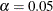and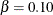. The null hypothesis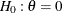and an alternative reference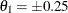are used for the designs with early stopping only to reject the null hypothesis.

#### Canonical Joint Distribution

The SEQDESIGN procedure assumes that with a total number of stages K, the sequence of the standardized test statistics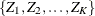has the canonical joint distribution with information levels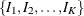for the parameter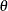(Jennison and Turnbull 2000, p. 49):

•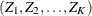is multivariate normal

•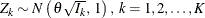•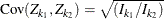,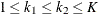#### Normality Assumption

The SEQDESIGN procedure derives the boundary values by assuming that the sequence of the standardized test statisticshas the canonical joint distribution with information levelsfor the parameter. If the test statistic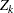does not have a normal distribution, it is assumed that the test statistic is computed from a large sample, so that the resulting statistic has an approximately normal distribution.

#### Number of Stages

For group sequential trials with fixed significance level, power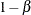, and alternative reference, if the number of stages is increased, the required maximum information is also increased, but the average sample number under the alternative hypothesis is likely to decrease.

For example, for two-sided designs with early stopping only to reject the null hypothesis,, andat the alternative reference, the O’Brien-Fleming method increases the maximum information from 168.12 for a fixed-sample design to 169.32 for a two-stage design, 172.57 for a five-stage design, and then 174.42 for a ten-stage design. In the mean time, the average sample number (as a percentage of fixed-sample) under the alternative hypothesis decreases from 100 for a fixed-sample design to 85.11 for a two-stage design, 75.03 for a five-stage design, and then 71.80 for a ten-stage design. The reduction in average sample number decreases as the number of stages increases. Thus there seems to be little to gain from choosing a design with more than five stages (Pocock 1982, p. 155).

#### Alternative Reference

The alternative reference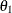is the hypothetical reference under the alternative hypothesis at which the power is computed. It is a treatment value that the investigators would hope to detect with high probability (Jennison and Turnbull 2000, p. 21).

For a group sequential design with specified parameters such asand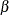errors, the drift parameter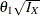is always derived in the SEQDESIGN procedure. Thus, with a smaller alternative reference, a larger maximum information level
is needed. That is, in order to detect a smaller difference with the same high power, a larger sample size is required.

#### Maximum Information

In a clinical trial, the amount of information about an unknown parameter available from the data can be measured by the Fisher information, the variance of the score statistic. The maximum information is the information level needed at the final stage of the group sequential trial if the trial does not stop at an interim stage. For a group sequential design, the maximum information can be derived with the specified alternative reference.

The maximum information is proportional to the sample size or number of events required for the design. Thus, it can also be used to compare different designs. Generally, a design with a larger probability to stop the trial early tends to have a larger maximum information. For example, for two-sided four-stage designs with early stopping only to reject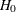,, andat the alternative reference, the Pocock method has a maximum information of 198.91 and the O’Brien-Fleming method has a maximum information of 171.84, indicating a much larger information level required for the Pocock method.

#### Drift Parameter

The drift parameteris derived for each design in the SEQDESIGN procedure, whereis the alternative reference. It is proportional to the square root of maximum information required for the design and can be used to compare maximum information for different designs with the same alternative reference. For example, for two-sided four-stage designs with early stopping only to reject,, and, the Pocock method has a drift parameter 3.526 and the O’Brien-Fleming method has a drift parameter 3.277, indicating that a larger maximum information level is required for the Pocock method than for the O’Brien-Fleming method.

#### Average Sample Number

The average sample number is the expected sample size (for nonsurvival data) or expected number of events (for survival data) of the design under a specific hypothetical reference. The percent average sample numbers with respect to the corresponding fixed-sample design are displayed in the SEQDESIGN procedure.

The design that requires a larger maximum information level tends to have a smaller average sample number under the alternative hypothesis. For example, for two-sided four-stage designs with early stopping only to reject,, andat the alternative reference, the Pocock design has a maximum information of 198.91 and an average sample number (in percentage of fixed-sample design) of 69.75 under the alternative hypothesis, and the O’Brien-Fleming design has a maximum information of 171.84 and an average sample number of 76.74.

#### Sample Size

The maximum information for the sequential design expressed as a percentage of its corresponding fixed-sample information is derived in the SEQDESIGN procedure. The sample size or number of events needed for a group sequential trial is computed by multiplying the sample size or number of events for the corresponding fixed-sample design by the derived percentage.

If the sample size or number of events for the fixed-sample design is available, you can use the MODEL=INPUTNOBS or MODEL=INPUTNEVENTS option in the SAMPLESIZE statement to derive the sample size or number of events needed at each stage. Otherwise, with the specified or derived maximum information, you can use the MODEL= option in the SAMPLESIZE statement to specify a hypothesis test and then to derive the sample size or number of events needed at each stage. See the section Sample Size Computation for the sample size computation for commonly used tests.